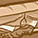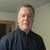Announcements

# AURIX™ Forum DiscussionsLevel 3
Level 3# How to calculate EVADC conversion time

Hello,

I am having trouble calculating EVADC conversion time, how can I know the frequency used by adc, in the clocking system chapter it is mentioned that fadc = fsource1, is fsource1 same as fpll1? if so I have fpll1 =300Mhz, so is fadc now 300MHZ and fadci = fadc/(DIVA+1)?

it seems something in my understanding is incorrect so please correct me.
4 RepliesEmployee
Employee# Re: How to calculate EVADC conversion time

I would expect that you would configure PLL1 to be set to 320MHz and then bit CCUCON1.PLL1DIVDIS=0 so you would get a divide by 2 for fSOURCE1 (160MHz). The maximum input clock frequency for the EVADC is 160MHzLevel 3
Level 3# Re: How to calculate EVADC conversion time

and what is NCR in the conversion time formula, how can I get this configured value?Employee
Employee# Re: How to calculate EVADC conversion time

TC37xx wrote:
and what is NCR in the conversion time formula, how can I get this configured value?

I am not sure where NCR is coming from. Looking at the users manual under section 32.9.2 Standard Convert Channels Timing:
tC12 = [(2 + STC) × tADCI] + [13 × tADCI] + [NRS × tNR] + [tPC] + [3 × tADC]
with:
tNR = 4 × tADCI + 3 × tADC per conversion step
tPC = (4 + 2×CALSTC) × tADCI + 5 × tADC if enabled, otherwise 0Level 3
Level 3# Re: How to calculate EVADC conversion time

I am having some trouble with calculating the conversion time, I have now derived all the required parameters to substitute in the conversion time formula, however the result I am getting is far from what I observe if I calculate the conversion time with STM.

Fosc is configured to be 20Mhz. NDIV+1 and PDIV+1 in PERPLLCON as 28 and 1, KDIV2+1 is 5. so I derived from the following Fpll1 = 112Mhz, and from CCUCON1.PLL1DIVDIS=1 and CLKSEL =01, Fsource1=fpl11, so Fsource1= Fadc =112 MHz, is there something wrong here ?

Step 2
STC=256